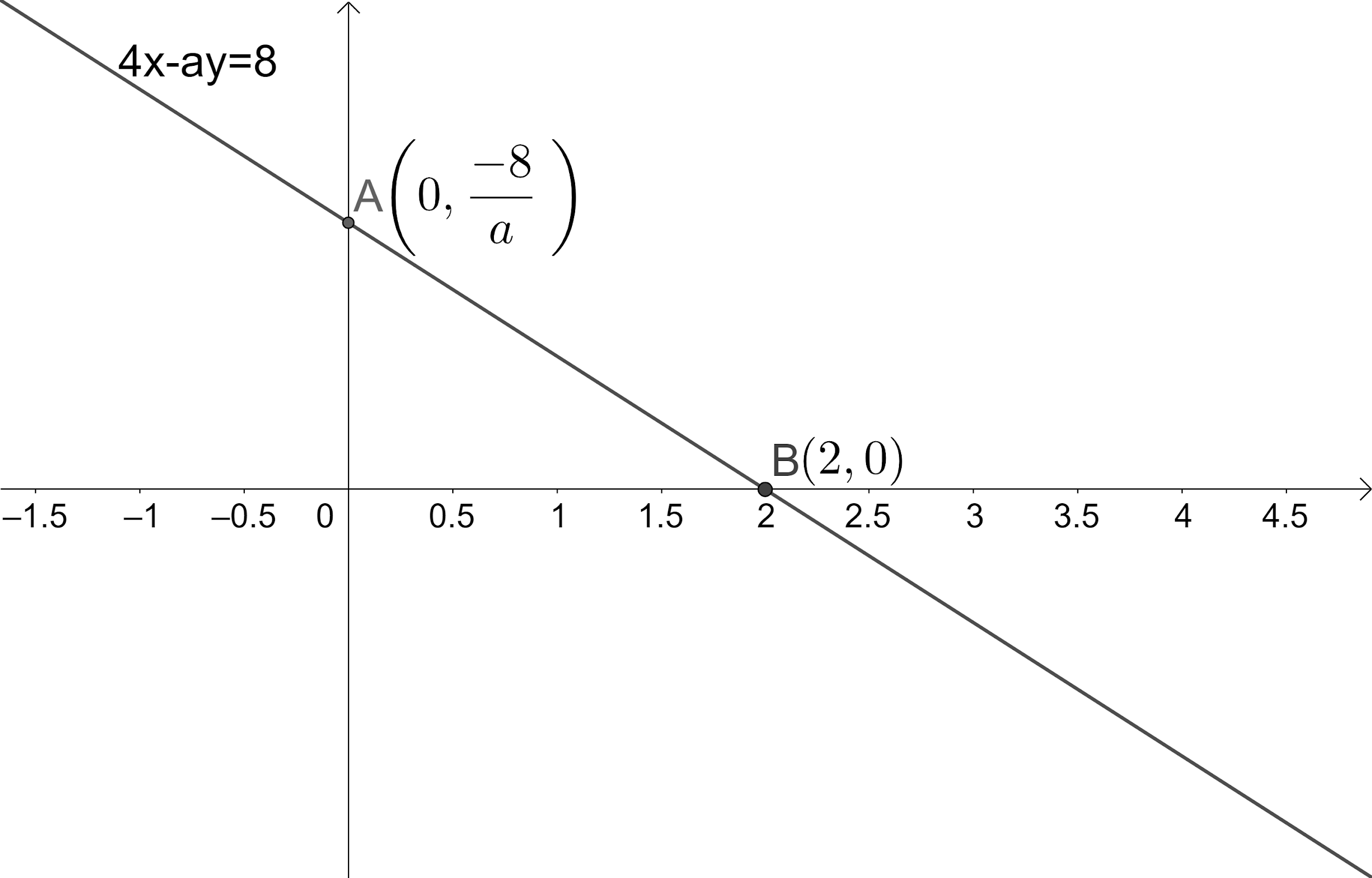QuestionAnswers

# If the y-intercept of the line 4x – ay = 8 is thrice of its x-intercept, then find the value of ‘a’?(a) $\dfrac{3}{4}$,(b) $\dfrac{4}{3}$,(c) $-\dfrac{3}{4}$,(d) $-\dfrac{4}{3}$,(e) $-\dfrac{2}{3}$.

Hint: We start solving the problem by recalling the intercept form of the equation of the line. We convert the given equation of line into the intercept form to find x and y-intercepts. Once we find them, we use the relationship between x and y-intercepts in the problem to get the required value of a.

Given that the value of y-intercept of the line 4x – ay = 8 is thrice the value of its x-intercept. We need to find the value of ‘a’.
Let us draw the line showing its intercepts.We know that the equation of the line showing x and y-intercepts are of the form $\dfrac{x}{a}+\dfrac{y}{b}=1$. Where x-intercept is a and y-intercept is b.
Let us convert the given equation of the line 4x – ay = 8 into the form that shows both x and y intercept.
We have the equation of the line as 4x – ay = 8.
$\Rightarrow \dfrac{4x-ay}{8}=\dfrac{8}{8}$.
$\Rightarrow \dfrac{x}{2}-\dfrac{ay}{8}=1$.
$\Rightarrow \dfrac{x}{2}+\dfrac{y}{\dfrac{-8}{a}}=1$.
$\Rightarrow \dfrac{-8}{a}$.
According to the problem it is given that y-intercept is 3 times the x-intercept.
$\Rightarrow \dfrac{-8}{a}=3\times 2$.
$\Rightarrow \dfrac{-8}{a}=6$.
$\Rightarrow \dfrac{-8}{6}=a$.
$\Rightarrow a=\dfrac{-4}{3}$.
We have found the value of ‘a’ as $\dfrac{-4}{3}$.
∴ The value of ‘a’ is $\dfrac{-4}{3}$.

So, the correct answer is “Option D”.

Note: If we don’t know the intercept form of the equation of the line, we find the intersection points of line with both x and y axes. We find the value of intercepts by finding the distance between origin and the points that we have just found on the axes. We should know that the intercept of the line can be negative. In this problem since the given line has positive x-intercept the y-intercept must be taken positive.